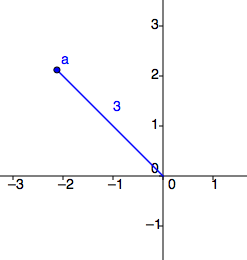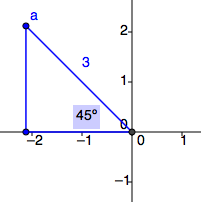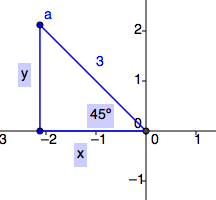### Home > PC > Chapter 11 > Lesson 11.1.1 > Problem11-17

11-17.

Given the polar point, find its location in the Cartesian coordinate system.

1. $(3, \frac { 3 \pi } { 4 })$

Graph the point.Create a right triangle with the x-axis.Find the lengths of the legs of the triangle. Use the lengths to determine the coordinates of point (a).1. $(−5, \frac { 3 \pi } { 2 })$